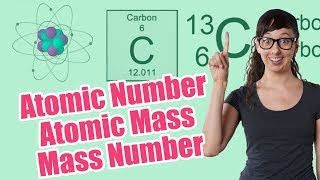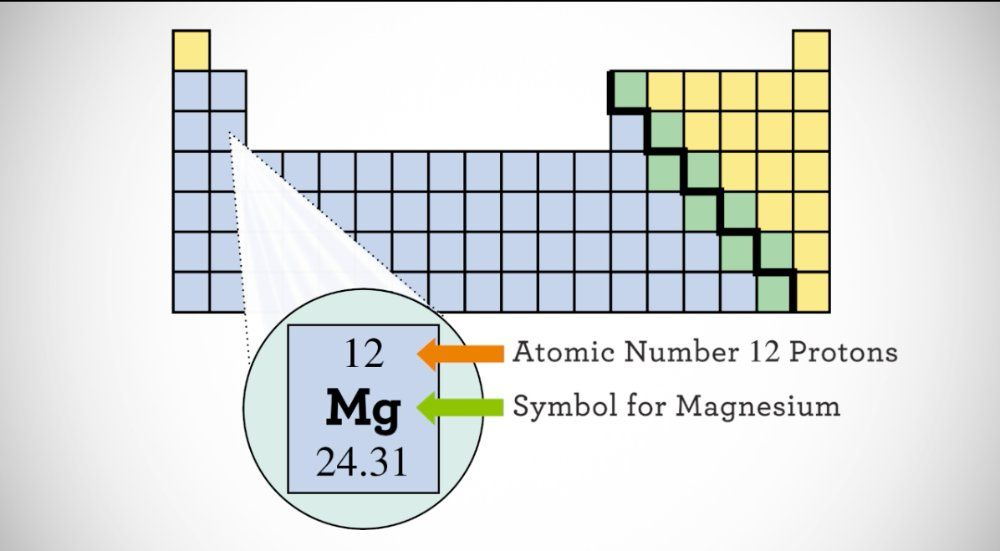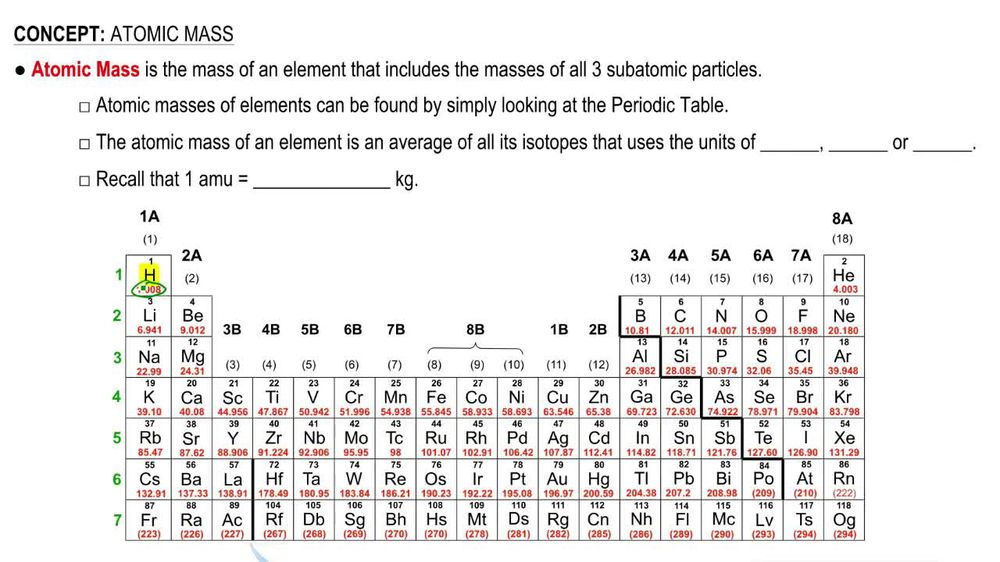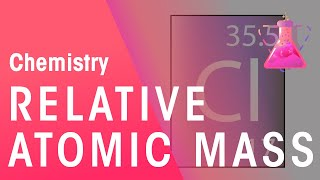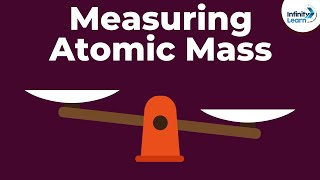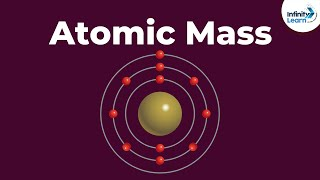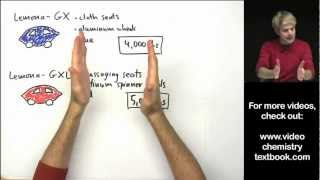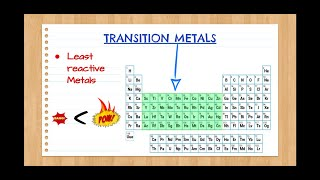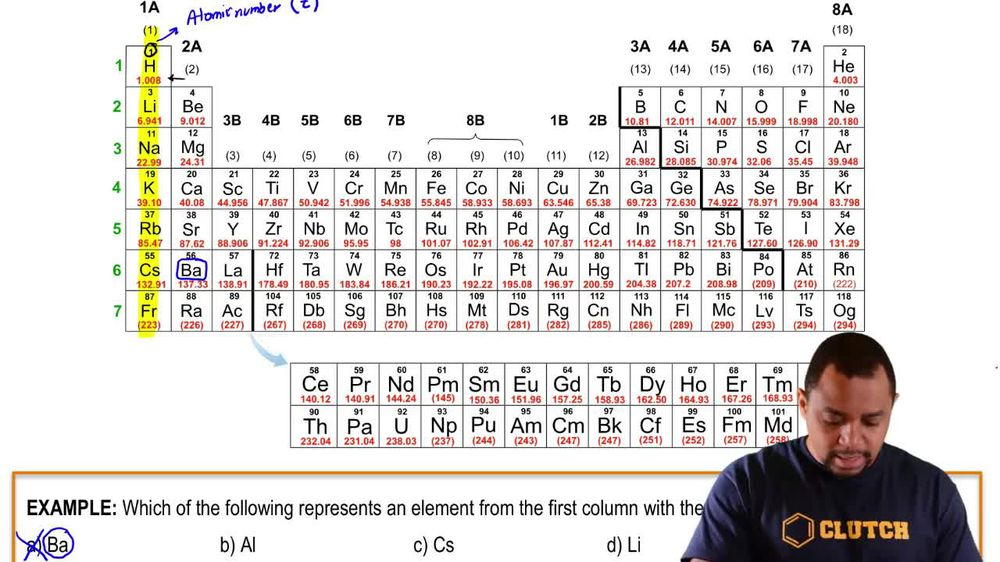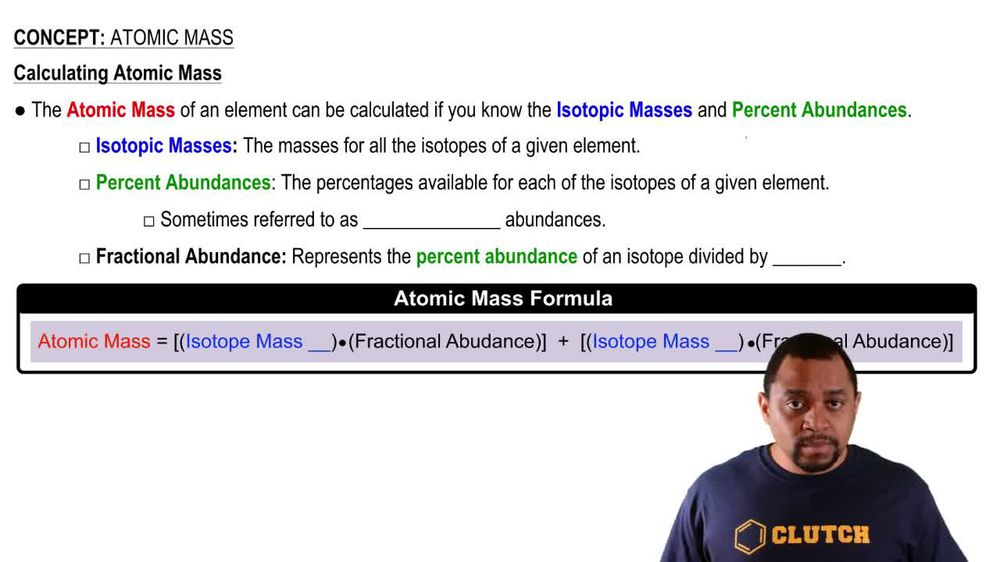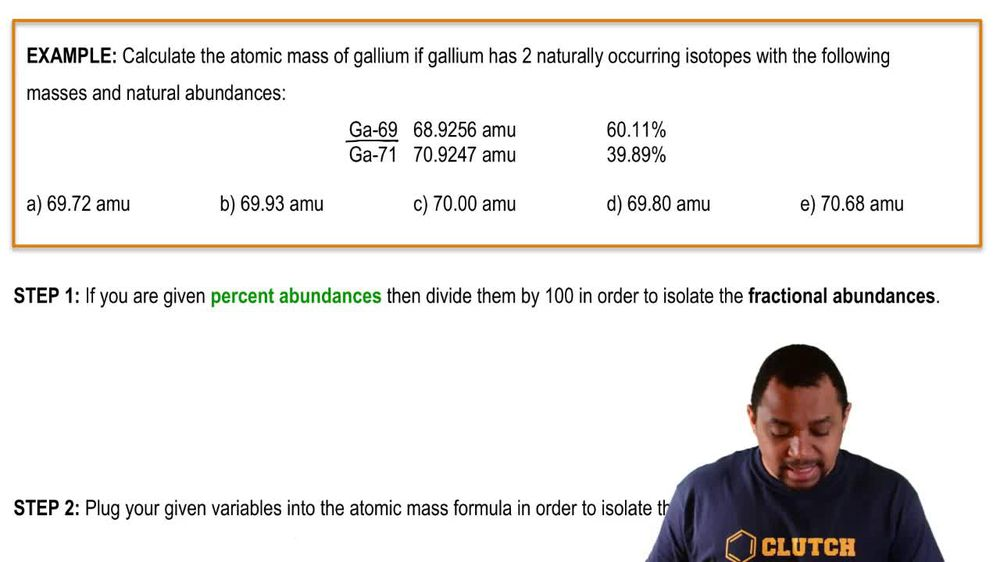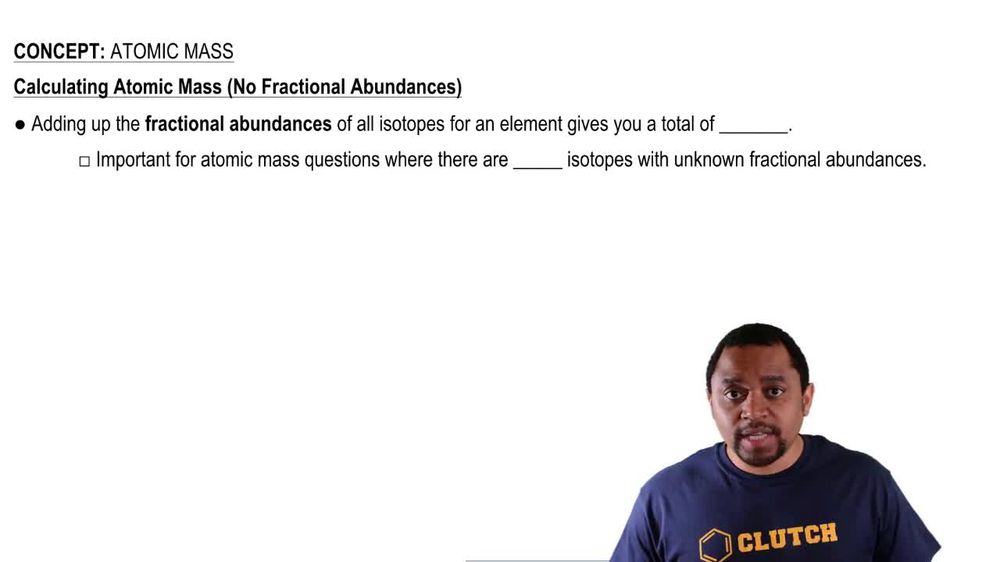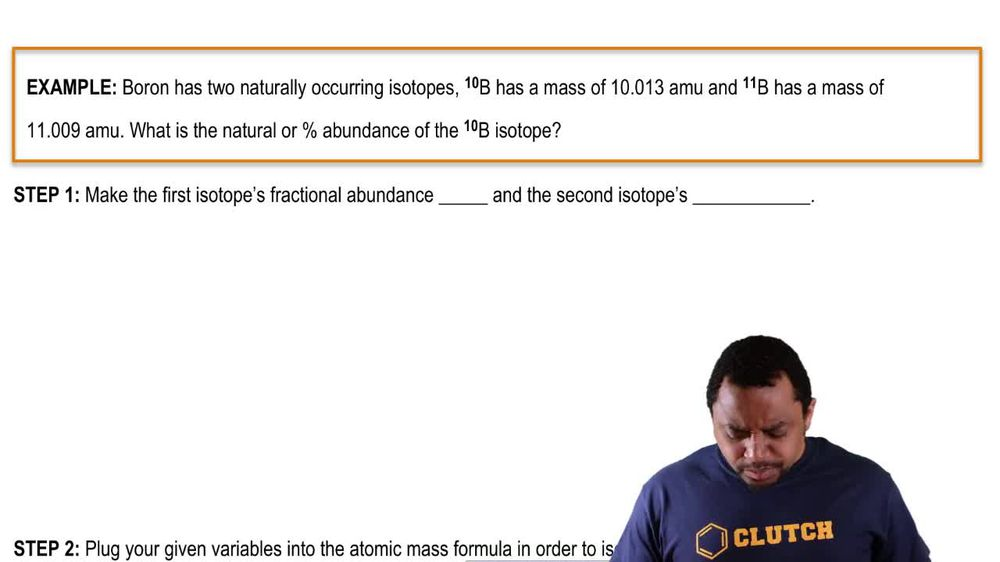Start typing, then use the up and down arrows to select an option from the list.
1. 2. Atoms & Elements2. Atomic Mass
Problem

# An element has four naturally occurring isotopes with the masses and natural abundances given here. Find the atomic mass of the element and identify it. Isotope Mass (amu) Abundance (%) 1 135.90714 0.19 2 137.90599 0.25 3 139.90543 88.43 4 141.90924 11.13

Relevant Solution2m
Play a video:
hey everyone in this example we have one isotope of an element with the following mass and the following percent abundance. And we have another isotope with the Mass of 70.92, am use. And we need to identify the identity of this element and its atomic mass. So what we're gonna do is recall that atomic mass is equal to our atomic mass of our isotope one multiplied by its relative abundance. And then this is going to be divided by our and let's just place this in a fraction divided by our atomic mass of isotope too, multiplied by its relative abundance. And so what we're going to have is that our percent abundance of isotope too Is going to equal 100% -60.11%. And this gives us a difference of 39.89%. So we can convert these two decimals and state that are relative abundance of isotope one is equal to 60.11 to a decimal as 0.6011. And then the relative abundance of isotope two equal to 39.89% as a decimal 0.3989. And so we can find our atomic mass of this element by taking 68.92, Am use which is the the given mass of our first isotope and multiplying it by our decimal here. So multiplying it by 0.60 11 of its relative abundance. And then we're going to divide this by our given mass of the second isotope 70.92 47 AM use and multiplying it by its relative abundance As a decimal 0.3989. And this gives us our atomic mass equal to 69.723 am use. And so we would actually refer to our paradox tables and see that this corresponds to the atom gallium G. A. And so this would complete this example as our final answer because we were able to identify identify its atomic mass and the identity of this gallium isotope. So if you have any questions, please leave them down below and I will see everyone in the next practice video.